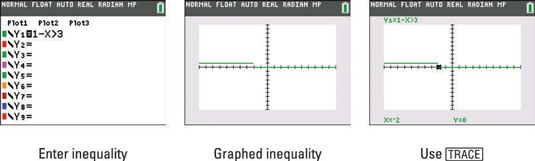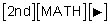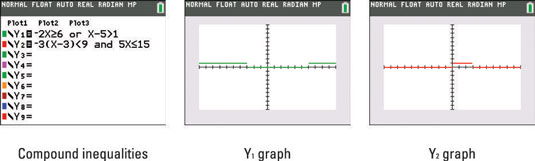##### TI-84 Plus CE Graphing Calculator For Dummies, 3rd EditionYour TI-84 Plus calculator was not made to graph inequalities on a number line, but it can be used to accomplish that task. The reason your calculator is able to perform a task that it was not designed for is the Boolean logic your calculator uses to evaluate statements.

You may remember that your calculator uses truth values: 1 = True and 0 = False.

When you enter a statement like 1 – X > 3, your calculator figures out where the statement is true and returns a 1, and where the statement is false returns the value of 0. Why not use this to your advantage in a graphing environment?

Follow these steps to graph a one-variable inequality (as if graphing on a number line) on your calculator:

1. Press [Y=] and enter the entire inequality.

See the first screen.

Press [2nd][MATH] to enter an inequality from the Test menu.2. Press [ZOOM] to graph the one-variable inequality.

See the second screen. When the y-value of the graph is 1, the inequality is true. When the y-value of the graph is 0, the inequality is false.

Your graph looks like a number line. The number line is slightly above the x-axis in a similar way that my students like to draw a number line on their homework. What is the only thing that is missing? Is the point at x = –2 opened or closed? See the next step for a method of checking the truth value at x = –2.

3. Press [TRACE] and enter an x-value you would like to check.

See the third screen. Entering –2 yields a y-value of 0 (which means false). You can safely conclude the point is open at x = –2 and the solution inequality is x < –2.

Press [2nd][GRAPH] to display the table. This is a really interesting way of looking at the truth values (of 1 and 0) that are returned for the inequality you enter.

This technique works for compound inequalities as well. The only drawback is that it can be difficult to determine the exact value where the graph begins.

The steps for entering compound inequalities are exactly the same as entering one-variable inequalities. In the first screen, an “or” inequality is entered for Y1 and an “and” inequality for Y2. Y1 is graphed in the second screen and Y2 in the third screen.

Insert “and” or “or” from the Logic menu and press